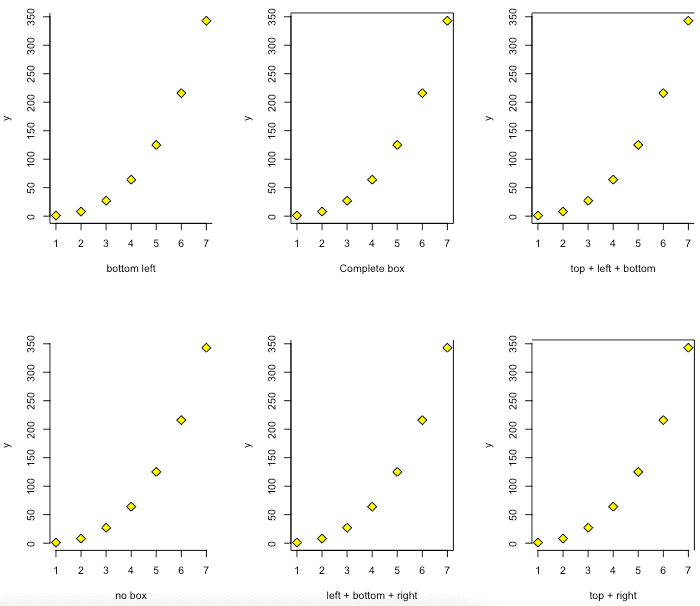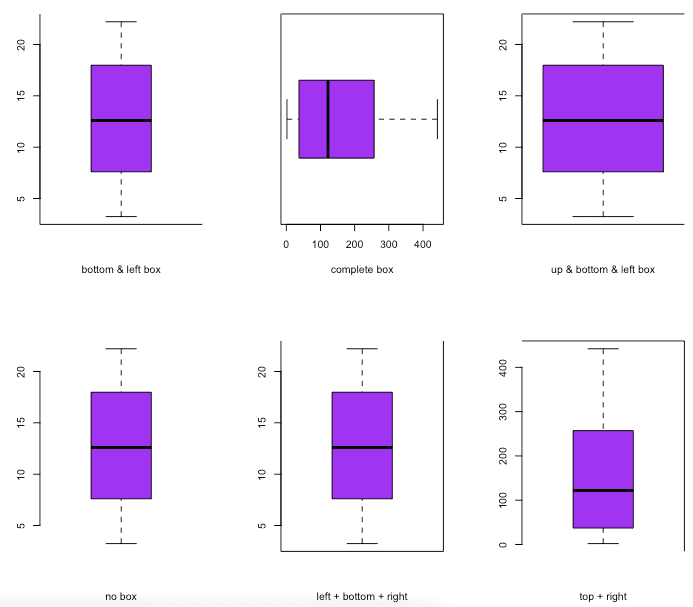# bty in R: What is Control box type in R

The bty option in the R plot() function controls the box style of the base. The bty is a parameter of the par() method that allows the box’s custom around the plot. The shape of the letter represents the boundaries.

1. o: complete box (default parameter),
2. n: no box
3. 7: top + right
4. L: bottom + left
5. C: top + left + bottom
6. U: left + bottom + right

### Example

``````par(mfrow = c(2, 3))

# Create data
x <- c(1, 2, 3, 4, 5, 6, 7)
y <- c(1, 8, 27, 64, 125, 216, 343)

# First graph
par(bty = "l")
plot(x, y, pch = 23, col = "black", bg = "yellow", lwd = 1, cex = 1.5, xlab = "bottom left")

# Second graph
par(bty = "o")
plot(x, y, pch = 23, col = "black", bg = "yellow", lwd = 1, cex = 1.5, xlab = "Complete box")

# Third graph
par(bty = "c")
plot(x, y, pch = 23, col = "black", bg = "yellow", lwd = 1, cex = 1.5, xlab = "top + left + bottom")

# Fourth graph
par(bty = "n")
plot(x, y, pch = 23, col = "black", bg = "yellow", lwd = 1, cex = 1.5, xlab = "no box")

# Fifth graph
par(bty = "U")
plot(x, y, pch = 23, col = "black", bg = "yellow", lwd = 1, cex = 1.5, xlab = "left + bottom + right")

# Sixth graph
par(bty = "7")
plot(x, y, pch = 23, col = "black", bg = "yellow", lwd = 1, cex = 1.5, xlab = "top + right")``````

OutputThe bty option of the par() function allows customizing the box around the plot.

To plot a chart of a function in R, you can use the plot() function. The plot() is a built-in method for plotting R objects. The bty parameter determines the type of box drawn. Then we plotted a graph using six different box types.

## Using boxplot() function with bty argument

Boxplots measure how well the data is distributed in the data set.

It divides the data set into three quartiles. This graph represents the data set’s minimum, maximum, median, first quartile, and third quartile.

``````par(mfrow = c(2, 3))

# Create data
a <- seq(1, 21) + 3 * runif(21, 0.3)
b <- seq(1, 21) ^ 2 + runif(21, 0.98)

# First graph
par(bty = "l")
boxplot(a, col = "purple", xlab = "bottom & left box")

# Second graph
par(bty = "o")
boxplot(b, col = "purple", xlab = "complete box", horizontal = TRUE)

# Third graph
par(bty = "c")
boxplot(a, col = "purple", xlab = "up & bottom & left box", width = 0.5)

# Fourth graph
par(bty = "n")
boxplot(a, col = "purple", xlab = "no box")

# Fifth graph
par(bty = "U")
boxplot(a, col = "purple", xlab = "left + bottom + right")

# Sixth graph
par(bty = "7")
boxplot(b, col = "purple", xlab = "top + right")
``````

OutputThat is it for the bty in R tutorial.

lwd in R

pch in R

Scatterplot in R

### 2 thoughts on “bty in R: What is Control box type in R”

1.Omigosh!!!!

2.